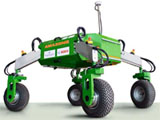## Biological Systems Engineering## Biological Systems Engineering: Papers and Publications

2017

#### Citation

Transactions of the ASABE Vol. 60(3): 693-701 (2017)

https://doi.org/10.13031/trans.12121

Copyright 2017 ASABE; used by permission.

#### Abstract

Five models for estimating fuel consumption for agricultural tractors with partial drawbar loads were compared. Data were collected from eight John Deere tractors, JD 7230R (e23), 7250R (e23), 7270R (e23), 7290R (e23), 8320R (16 speed), 7290R (IVT), 8345RT (IVT), 8370R (IVT), on the drawbar test track at the Nebraska Tractor Test Lab. The tractors were tested with seven load levels per speed at three different travel speeds as close as possible to 7.5, 10, and 13 km h-1. The IVT tractors were operated in auto mode, and the geared tractors were shifted up three gears and throttled back to the same travel speeds as obtained with the original gear (before shifting up) at maximum drawbar power. The seven loads were selected at 30%, 40%, 50%, 60%, 70%, 75%, and 80% of the drawbar pull at maximum power and rated engine speed at the selected travel speed. Model 1 (fuel consumption as a linear function of drawbar power on concrete), currently used in OECD Code 2, Section 4.4.8, resulted in a separate equation for each speed tested. When regression mean square errors were used for statistical comparison of the five fuel consumption models, model 5 (fuel consumption as a linear function of drawbar power and travel speed on concrete, and engine speed) was not significantly different from the model currently used in OECD Code 2, Section 4.4.8 (model 1, fuel consumption as a linear function of drawbar power on concrete, with separate equations specific to the three speeds tested). The simplest model (model 2), which used a single equation for fuel consumption as a linear function of drawbar power on concrete over the range of speeds tested, had significantly higher regression mean square errors compared to model 1 for half of the eight tractors tested. Model 5 (fuel consumption as a linear function of drawbar power and travel speed on concrete, and engine speed) was determined to be the best of the five models for estimating fuel consumption, with a single equation applicable over the range of speeds tested. Model 3 (fuel consumption as a linear function of drawbar power and travel speed on concrete) provided a statistically equivalent fuel consumption estimate to model 5 without the drawback of requiring an input value for engine speed.

COinS